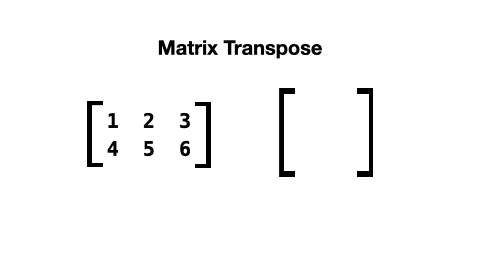A matrix transpose creates a flipped version of the matrix and can be done easily in Python. In this example, you see a list comprehension that transposes a matrix.

# Matrix transpose in Python.

When a matrix is transposed, its rows and columns are switched like this:The list comprehension to do this looks like this:

```def transpose_matrix(matrix):
return [[row[col] for row in matrix] for col, _ in enumerate(matrix)]```

The matrix should have a rectangular form. All rows should be of equal size and all columns should be of equal size.

Here is a test program that uses the transpose function:

```matrix = [
[1, 2, 3],
[4, 5, 6]
]

def print_matrix(matrix):
for row in matrix:
print(" ".join([str(col) for col in row]))
print()

def transpose_matrix(matrix):
return [[row[col] for row in matrix] for col, _ in enumerate(matrix)]

print_matrix(matrix)
transposed_matrix = transpose_matrix(matrix)
print_matrix(transposed_matrix)
transposed_again_matrix = transpose_matrix(transposed_matrix)
print_matrix(transposed_again_matrix)```

Output:

```1 2 3
4 5 6

1 4
2 5
3 6

1 2 3
4 5 6```
Written by Loek van den Ouweland on 2021-08-20.
Questions regarding this artice? You can send them to the address below.